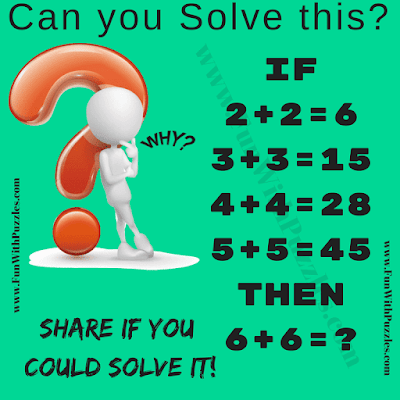Here is a very interesting Mathematical Puzzle for Teens. Here we are given Puzzle Picture which contains the logical statements. These statements are represented by Mathematical equations. Your challenge is to decode these equations and then find the value of missing number which will replace the question mark. Let's see if you can solve this Mathematical Puzzle?Can you solve this Mathematical Puzzle?

The answer to this "Mathematical Puzzle for Teens", can be viewed by clicking on the button. Please do give your best try before looking at the answer.